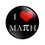# Stuck!

Here is a problem from the NMTC 2015 Bhaskara Contest:

$\text{The number of values of}\space x\space \text{which satisfy the equation}\space 5^2.\sqrt[x]{8^{x-1}}=500\space \text{are:}$

Here is the link. (Question 6).

I solved it like this:

$5^2.\sqrt[x]{8^{x-1}}=500$

$\Rightarrow\space 5^2.(8^{x-1})^{\frac{1}{x}}=2^2\times5^3$

$\Rightarrow\space 5^2.8^{\frac{x-1}{x}}=(2^3)^{\frac{2}{3}}\times5^3$

$\Rightarrow\space 8^{\frac{x-1}{x}}=8^{\frac{2}{3}}\times5$

But here, I am stuck. Any idea?Note by Aaryan Maheshwari
4 years ago

This discussion board is a place to discuss our Daily Challenges and the math and science related to those challenges. Explanations are more than just a solution — they should explain the steps and thinking strategies that you used to obtain the solution. Comments should further the discussion of math and science.

When posting on Brilliant:

• Use the emojis to react to an explanation, whether you're congratulating a job well done , or just really confused .
• Ask specific questions about the challenge or the steps in somebody's explanation. Well-posed questions can add a lot to the discussion, but posting "I don't understand!" doesn't help anyone.
• Try to contribute something new to the discussion, whether it is an extension, generalization or other idea related to the challenge.

MarkdownAppears as
*italics* or _italics_ italics
**bold** or __bold__ bold
- bulleted- list
• bulleted
• list
1. numbered2. list
1. numbered
2. list
Note: you must add a full line of space before and after lists for them to show up correctly
paragraph 1paragraph 2

paragraph 1

paragraph 2

[example link](https://brilliant.org)example link
> This is a quote
This is a quote
    # I indented these lines
# 4 spaces, and now they show
# up as a code block.

print "hello world"
# I indented these lines
# 4 spaces, and now they show
# up as a code block.

print "hello world"
MathAppears as
Remember to wrap math in $$ ... $$ or $ ... $ to ensure proper formatting.
2 \times 3 $2 \times 3$
2^{34} $2^{34}$
a_{i-1} $a_{i-1}$
\frac{2}{3} $\frac{2}{3}$
\sqrt{2} $\sqrt{2}$
\sum_{i=1}^3 $\sum_{i=1}^3$
\sin \theta $\sin \theta$
\boxed{123} $\boxed{123}$

Sort by:

Actually, this is my father's account, and now I use it. I am in 7th standard. So please can you explain what 'log' is or explain without log?😊

- 4 years ago

Check out the Brilliant wiki to learn logarithms which is pretty good.

- 4 years ago

Sir, you can continue from your approach by dividing both sides by $8^{\frac{2}{3}}$ (in your final equation) and the applying log to the base $10$ on both sides(A one step (log rules) simplification is required). This equation is obviously a linear equation, thus having only one solution.

- 4 years ago

There is no solution of this equation. if have any give that soln

- 3 years, 11 months ago

It has no integral but a real sol

- 3 years, 11 months ago

Yep you are right buddy! It has no integral solutions but one real solution. So the answer is 1 as the question does not specify whether they should be integers or real number.

- 3 years, 11 months ago